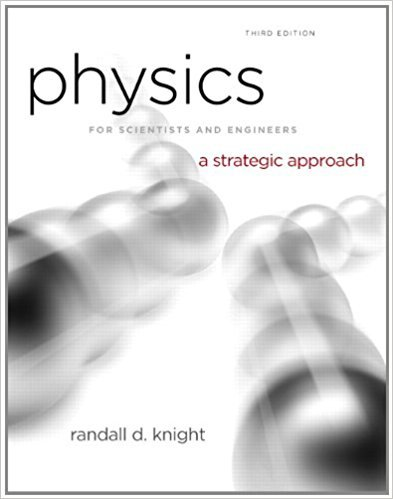×
Get Full Access to Physics For Scientists And Engineers: A Strategic Approach With Modern Physics - 3 Edition - Chapter 14 - Problem 19e
Get Full Access to Physics For Scientists And Engineers: A Strategic Approach With Modern Physics - 3 Edition - Chapter 14 - Problem 19e

×

# A mass on a string of unknown length oscillates as aISBN: 9780321740908 69

## Solution for problem 19E Chapter 14

Physics for Scientists and Engineers: A Strategic Approach with Modern Physics | 3rd Edition

• Textbook Solutions
• 2901 Step-by-step solutions solved by professors and subject experts
• Get 24/7 help from StudySoup virtual teaching assistantsPhysics for Scientists and Engineers: A Strategic Approach with Modern Physics | 3rd Edition

4 5 1 373 Reviews
23
4
Problem 19E

Problem 19E

A mass on a string of unknown length oscillates as a pendulum with a period of 4.00 s. What is the period if

a. The mass is doubled?

b. The string length is doubled?

c. The string length is halved?

d. The amplitude is halved?

Parts a to d are independent questions, each referring to the initial situation.

Step-by-Step Solution:

Solution 19 E:

Step 1 of 1:-

The oscillation time period is given as,.

The mass is m.

The length of the string is.

a)

If the mass is doubled, means, then what will be the time period.

We know that,------------(1)

Where g is the acceleration due to gravity.

The above equation is independent of the mass attached with the string.

So, when the mass is doubled, the time period will remain same as 4 s.

b)

When the string length is doubled,.

So, the time period will become 5.65 s.

c)

When the string length is halved,.

So, the time period will become 2.82 s.

d)

When the amplitude is halved, the time period will be invariant. Because it is independent of the amplitude.

But if the angleincreases more and we cannot take the approximation ofanymore, then this formula will not hold.

Step 2 of 1

##### ISBN: 9780321740908

Unlock Textbook Solution